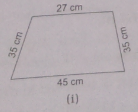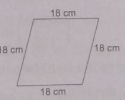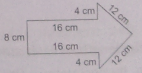RS Aggarwal Class 6 Solutions Chapter 21 - Concept Of Perimeter And Area

RS Aggarwal Class 6 Chapter 21 - Concept Of Perimeter And Area Solutions Free PDF

In this chapter, the students will learn the basic concepts of area and perimeter. Before solving the questions let us understand the terms perimeter and area.
Perimeter: Perimeter is the path around a two-dimensional shape.
Area: The area of a plane figure or of a flat surface is the number of unit squares that can be contained within it.

Learn the concepts of area and perimeter by solving questions from RS Aggawaral for class 6. For solutions to the questions, students can also refer to the RS Aggarwal class 6 solutions chapter 21 concept of perimeter and area.

1. Find the perimeter of a rectangle in which:

(i) Length = 16.8 cm and breadth = 6.2 cm

Length = 16.8 cm

Perimeter of a rectangle = $2 \times \left ( length + breadth \right )$

= $2 \times \left ( 16.8 + 6.2 \right ) = 46 cm$

(ii) Length = 2 m 25 cm and breadth = 1 m 50 cm

Length = 2 m 25 cm

= (200 + 25) cm (1 m = 100 cm)

= 225 cm

Breadth = 1 m 50 cm

= (100 + 50) cm (1 m = 100 cm)

= 150

Perimeter of a rectangle = $2 \times \left ( length + breadth \right )$

= $2 \times \left ( 225 + 150 \right ) = 750 cm$

(iii) Length = 8 m 5 dm and breadth = 6 m 8 dm

Length = 8 m 5 dm

= (80 + 5) dm (1 m = 10 dm)

= 85 dm

Breadth = 6 m 8 dm

= (60 + 8) dm (1 m = 10 dm)

= 68 dm

Perimeter of a rectangle = $2 \times \left ( length + breadth \right )$

= $2 \times \left ( 85 + 68 \right ) = 306 dm$

2. Find the cost of fencing a rectangular field 62 m long and 33 m wide at Rs 16 per meter.

Length of the field = 62 m

Breadth of the field = 33 m

Perimeter of a field = $2 \times \left ( length + breadth \right )$

= $2 \times \left ( 62 + 33 \right ) m = 190 m$

Cost of fencing per meter = Rs 16

Total cost of fencing = Rs (16 × 190) = Rs 3040

3. The Length and the breadth of a rectangle field are in the ration 5 : 3. If its perimeter is 128 m, find the dimensions of the field.

Let the length of the rectangle be 5x m.

Breadth of the rectangle = 3x m

Perimeter of a field = $2 \times \left ( length + breadth \right )$

= $2 \times \left ( 5x + 3x \right ) m = 16 m$

It is given that the perimeter of the field is 128 m.

Therefore, $16x = 128$

$\Rightarrow x = \frac {128} {16} = 8$

Therefore, $Length = \left ( 5 \times 8 \right ) = 40 m$

$breadth = \left ( 3 \times 8 \right ) = 24 m$

4. The cost of fencing the rectangle field at Rs 18 per metre is Rs 1980. If the width of the field is 23 m, find its length.

Total cost of fencing = Rs 1980

Rate of fencing = Rs 18 per meter

$Perimeter \; of \; the \; field = \frac {Total \; cost} {Rate} = \frac {Rs 1980} {Rs 18/m} = \left ( \frac {1980} {18} \right ) m = 110 m$

Let the length of the field be x metre.

Perimeter of a field = $2 \times \left ( x + 23 \right ) m$

Therefore, $2 \left ( x + 23 \right ) = 110$

$\Rightarrow \left ( x + 23 \right ) = 55$

$x = \left ( 55 – 23 \right ) = 32$

Hence, the length of the field is 32 m.

5. The length and the breadth of a rectangle field are in the ratio 7:4. The cost of fencing the field at Rs 25 per metre is Rs 3300. Find the dimension of the field.

Total cost of fencing = Rs 3300

Rate of fencing = Rs 25/m

$Perimeter \; of \; the \; field = \frac {Total \; cost} {Rate \; of \; fencing} = \left ( \frac {Rs \; 3300} {Rs \; 25/m} \right ) = \left ( \frac {3300} {25} \right ) m = 132 \; m$

Let the length and the breadth of the rectangular field be 7x and 4x, respectively.

Perimeter of the field = 2(7x + 4x) = 22x

It is given that the perimeter of the field is 132 m.

Therefore, $22x = 132$

$\Rightarrow x = \frac {132} {22} = 6$

Therefore, $Length \; of \; the \; field = \left ( 7 \times 6 \right ) m = 42 \; m$

$Breadth \; of \; the \; field = \left ( 4 \times 6 \right ) m = 24 \; m$

6. Find the perimeter of a square, each of whose sides measures:

(i) 3.8 cm

Side of the square = 3.8 cm

Perimeter of the square = (4 × side)

= ( 4 × 3.8 ) = 15.2 cm

(ii) 4.6 m

Side of the square = 4.6 m

Perimeter of the square = (4 × side)

= ( 4 × 4.6 ) = 18.4 m

(iii) 2 m 5 dm

Side of the square = 2 m 5 dm

= (20 + 5) dm (1 m = 10 dm)

= 25 dm

Perimeter of the square = (4 × side)

= ( 4 × 25 ) = 100 dm

7. The cost of putting a fence around a square field at Rs 35 per metre is Rs 4480. Find the length of each side of the field.

Total cost of fencing = Rs 4480

Rate of fencing = Rs 35/m

$Perimeter \; of \; the \; field = \frac {Total \; cost} {Rate } = \left ( \frac {Rs \; 4480} {Rs \; 35/m} \right ) = \left ( \frac {4480} {35} \right ) m = 128 \; m$

Let the length of the each side of the field be x metres.

Perimeter = (4x) metres

Therefore, $4x = 128$

$\Rightarrow x = \frac {128} {4} = 32$

Hence, the length of each side of the field is 32 m.

8. Each side of the square field measure 21 m. Adjacent to this field, there is a rectangular field having its sides in the ratio 4 : 3. If the perimeters of both the field are equal, find the dimensions of the rectangular field.

Side of the square field = 21 m

Perimeter of the square field = (4 × 21) m

= 84 m

Let the length and breadth of the rectangular field be 4x and 3x, respectively.

Perimeter of the rectangular field = Perimeter of the square field

Therefore, $14x = 84$

$\Rightarrow x = \frac {84} {14} = 6$

Therefore, Length of the rectangular field = $\left ( 4 \times 6 \right ) m = 24 \; m$

Breadth of the rectangular field = $\left ( 3 \times 6 \right )m = 18 \; m$

9. Find the perimeter of

(i) A rectangle of sides 7.8 cm, 6.5 cm and 5.9 cm

Sides of the triangle are 7.8 cm, 6.5 cm and 5.9 cm

Perimeter of a triangle = (First side + Second side + Third side)

(7.8 + 6.5 + 5.9) cm

= 20.2 cm

(ii) An equilateral triangle of side 9.4 cm

In an equilateral triangle, all sides are equal.

Length of each sides of the triangle = 9.4 cm

Therefore, Perimeter of the triangle = $\left ( 3 \times sides \right ) \;cm$

= (3 × 9.4) cm

= 28.2 cm

(iii) An isosceles triangle with equal sides 8.5 cm each and third side 7 cm.

Length of two equal sides = 8.5 cm

Length of the third side = 7 cm

Therefore, Perimeter of the triangle = $\left ( 2 \times equal \; sides \right ) + third \; side\; cm$

= {(2 × 8.5) + 7} cm

= 24 cm

10. Find the perimeter of

(i) A regular pentagon of side 8 cm

Length of each sides of the given pentagon = 8 cm

Therefore, perimeter of the pentagon = (5 × 8) cm

= 40 cm

(ii) A regular octagon of side 4.5 cm

Length of each sides of the given octagon = 4.5 cm

Therefore, perimeter of the octagon = (4.5 × 8) cm

= 36 cm

(iii) A regular decagon of side 3.6 cm

Length of each sides of the given decagon = 3.6 cm

Therefore, perimeter of the decagon = (10 × 3.6) cm

= 36 cm

10. Find the perimeter of each of the following figures:

(i)Perimeter of the figure = sum of all the sides

= (27 + 35 + 35 + 45) cm

= 142 cm

(ii)Perimeter of the figure = sum of all the sides

= (18 + 18 + 18 + 18) cm

= 72 cm

(iii)Perimeter of the figure = sum of all the sides

= (8 + 16 + 4 + 12 + 12 + 16 + 4) cm

= 72 cm

Exercise 21B

1. Find the circumference of the circle whose radius is

(i) 28 cm

Solution:

Therefore, Circumference of the circle, C = 2.pi.r

= $\left ( 2 \times \frac {22} {7} \times 28 \right )$

= 176 cm

Hence, the circumference of the given circle is 176 cm.

(ii) 10.5 cm

Solution:

Therefore, Circumference of the circle, C = 2.pi.r

= $\left ( 2 \times \frac {22} {7} \times 10.5 \right )$

= 66 cm

Hence, the circumference of the given circle is 66 cm.

(iii) 3.5 m

Solution:

Therefore, Circumference of the circle, C = 2.pi.r

= $\left ( 2 \times \frac {22} {7} \times 3.5 \right )$

= 22 m

Hence, the circumference of the given circle is 22 m.

2. Find the radius of the circle whose circumference is 176 cm.

Solution:

Let the radius of the given circle be r cm.

Circumference of the circle = 176 cm

Circumference = 2πr

Therefore, 2πr = 176

$\Rightarrow r = \frac {176} {2 \pi }$

$\Rightarrow r = \left ( \frac {176} {2} \times \frac {7} {22} \right )$

r = 28

The radius of the given circle is 28 cm.

4. Find the diameter of the wheel whose circumference is 264 cm

Solution:

Let the radius of the given circle be r cm.

Diameter = 2 × Radius = 2r cm

Circumference of the wheel = 264 cm

Circumference of the wheel = 2πr

Therefore, $2 \pi r = 264$

$\Rightarrow 2 r = \frac {264} {\pi }$

$\Rightarrow 2 r = \left ( 264 \times \frac {7} {22} \right )$

$\Rightarrow 2 r = 84$

Diameter of the given wheel is 84 cm.

5. Find the distance covered by the wheel of a car is 500 revolution if the diameter of the wheel is 77 cm.

Solution:

Radius of the wheel = (Diameter of the wheel)\2

$\Rightarrow r = \frac {77}{2}$

Circumference of the wheel = 2πr

= $\left ( 2 \times \frac {22} {7} \times \frac {77} {2} \right )$

= 242 cm

In 1 revolution the wheel covers a distance equal to its circumference.

Therefore, distance covered by the wheel in 1 revolution = 242 cm

Therefore, distance covered by the wheel in 500 revolution = (500 × 242) cm

= 121000 cm

= 1210 m (100 cm = 1 m)

= 1.21 km (1000 m = 1 km)

6. The diameter of the wheel of a car is 70 cm. how many revolutions will it make to travel 1.65 km?

Solution:

Radius of the wheel ( r ) = (Diameter of the wheel)\2

$r = \frac {70} {2} cm = 35 cm$

Circumference of the wheel = 2πr = $\left ( 2 \times \frac {22} {7} \times 35 \right )$

= 220 cm

In 1 revolution the wheel covers a distance equal to its circumference.

Therefore, 220 cm distance = 1 revolution

Therefore, 1 cm distance = 1/220 revolution

Therefore, $1 km \left ( 100000 \;cm \right ) = \frac {1 \times 100000} {220}\; revolutions$

Therefore, $1.65 \;km = \frac {1.65 \times 100000} {220}\; revolutions$

= 750 revolutions

Thus, the wheel will make 750 revolutions to travel 1.65 km

Practise This Question

For a magnet, its magnetic strength is equally distributed over its body.